Like   Tweet   Pin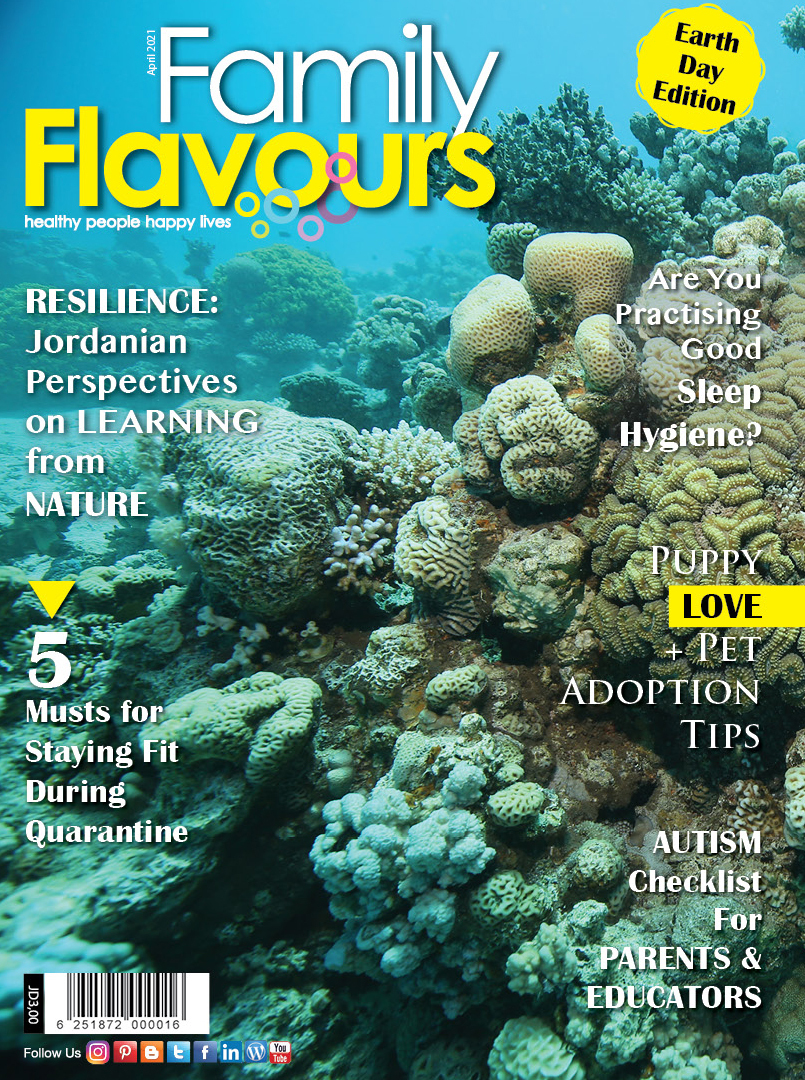Photo courtesy of the Royal Marine Conservation Society of Jordan (JREDS)

## On Our Cover: Corals (Platygyra daedalea) in the Gulf of Aqaba

RESILIENCE: Jordanian Perspectives on Learning from NATURE

Puppy LOVE + Pet Adoption Tips

Are You Practising Good Sleep Hygiene?

AUTISM Checklist For PARENTS & EDUCATORS

5 Musts for Staying Fit During Quarantine

 table div table+table+table div table{width:100%;padding:0}table div table+table+table div table img{width:96.23%;padding:0;float:none}table div table+table+table div table td{width:100%;padding:0 1.88% 18px}/* styles */## Resilience

Following the success of our Mind, Body, Soul IV virtual retreat on resilience last month, we explore what nature can teach us about strength, featuring perspectives from our panel of experts.Mohammad Tawaha, Marine Environment Researcher & Aqaba Office Manager at JREDS, delves deep to reveal the resilience of Aqaba’s coral reefs. He says we can “learn from the reefs that have survived for 485 million years as we aim to see ourselves through a pandemic and significant change to come”

 table div table+table+table+table+table+table div table{width:100%;padding:0}table div table+table+table+table+table+table div table img{width:96.23%;padding:0;float:none}table div table+table+table+table+table+table div table td{width:100%;padding:0 1.88% 18px}/* styles */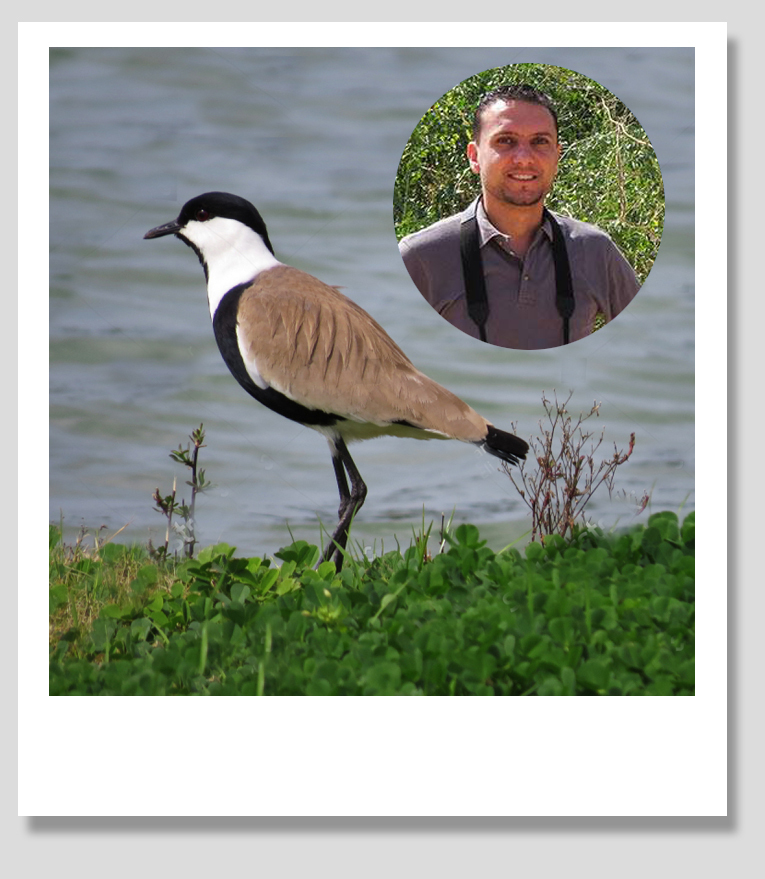Feras Rahahleh, Manager of Aqaba Bird Observatory, finds inspiration in the Spur-Winged Lapwing. “It is resilience that helps us cope, just like the innate resilience of other living creatures that helps them overcome challenges,” says Rahahleh

 table div table+table+table+table+table+table+table+table div table{width:100%;padding:0}table div table+table+table+table+table+table+table+table div table img{width:96.23%;padding:0;float:none}table div table+table+table+table+table+table+table+table div table td{width:100%;padding:0 1.88% 18px}/* styles */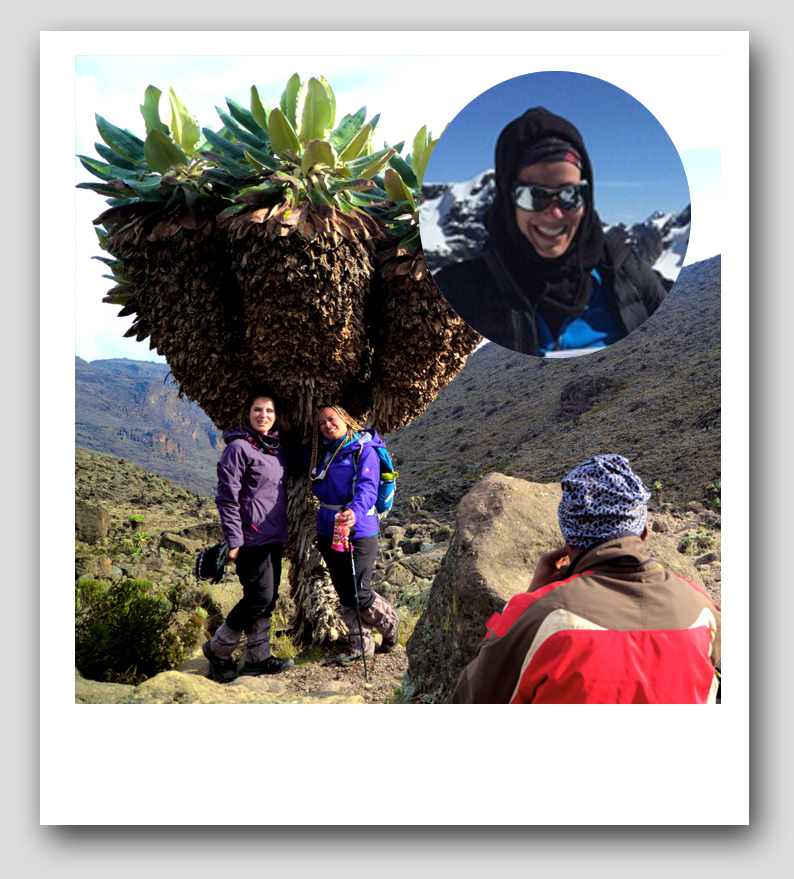Dr Sahar Jumean, Paediatric Dentist, finds inspiration in the world-renowned peaks she climbed in South America, Africa and South Asia and describes the resilience of the Dendrosenecio Kilimanjari; an unusual plant found only on Kilimanjaro.“This mysterious and unique plant reminded me that I, too, can be resilient in adapting to adversity and equally unbreakable,” she recalls

 table div table+table+table+table+table+table+table+table+table+table div table{width:100%;padding:0}table div table+table+table+table+table+table+table+table+table+table div table img{width:96.23%;padding:0;float:none}table div table+table+table+table+table+table+table+table+table+table div table td{width:100%;padding:0 1.88% 18px}/* styles */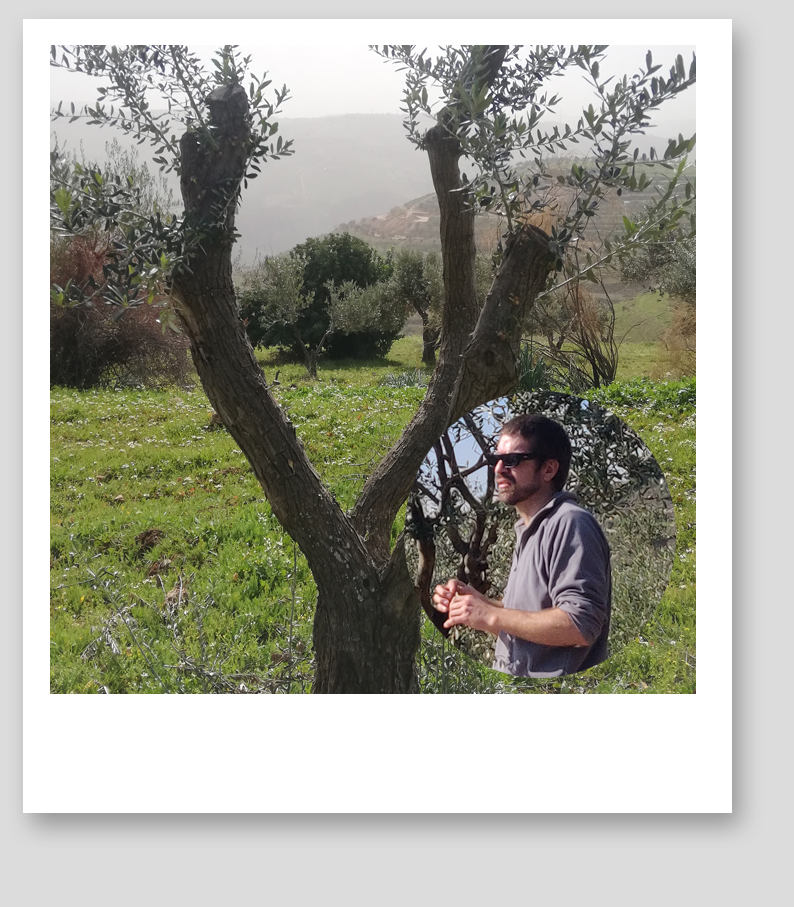Mu'taz Mango, producer of Al Yaqoota olive oil at Majdal Agricultural Company, finds inspiration in the olive tree. He shares with us the survival mechanisms that enable it to survive cold, heat, fire, disease and drought and the four key life skills we can learn from the olive tree

 table div table+table+table+table+table+table+table+table+table+table+table+table div table{width:100%;padding:0}table div table+table+table+table+table+table+table+table+table+table+table+table div table img{width:96.23%;padding:0;float:none}table div table+table+table+table+table+table+table+table+table+table+table+table div table td{width:100%;padding:0 1.88% 18px}/* styles */## Fit From Home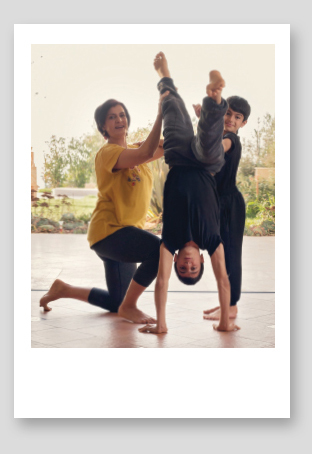▪ Yoga Instructor Rula Wardeh Sakkab dispenses advice for staying fit during self-quarantine
 ▪ Yoga Instructor Rula Wardeh Sakkab dispenses advice for staying fit during self-quarantine

▪ To get the kids moving on lockdown Fridays, UNICEF Kid Power is a free programme that features dance, yoga and learning videos: visit www.unicefkidpower.org
 ▪ To get the kids moving on lockdown Fridays, UNICEF Kid Power is a free programme that features dance, yoga and learning videos: visit www.unicefkidpower.org
 table div table+table+table+table+table+table+table+table+table+table+table+table+table+table+table div table{width:100%;padding:0}table div table+table+table+table+table+table+table+table+table+table+table+table+table+table+table div table img{width:96.23%;padding:0;float:none}table div table+table+table+table+table+table+table+table+table+table+table+table+table+table+table div table td{width:100%;padding:0 1.88% 18px}/* styles */## My Personal ExperiencePersonal Development Coach Abeer Jabaji, shares her experience of getting COVID-19 and what it taught her

 table div table+table+table+table+table+table+table+table+table+table+table+table+table+table+table+table+table+table div table{width:100%;padding:0}table div table+table+table+table+table+table+table+table+table+table+table+table+table+table+table+table+table+table div table img{width:96.23%;padding:0;float:none}table div table+table+table+table+table+table+table+table+table+table+table+table+table+table+table+table+table+table div table td{width:100%;padding:0 1.88% 18px}/* styles */## Supporting Children’s Wellbeing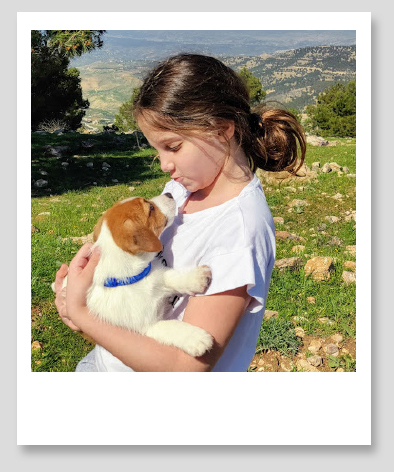Children are not immune from the toll that COVID-19 has been taking on our mental health.

We were inspired by one 10-year-old's insights on pets' positive effect on mental health – meet Ayla Haddad and her four-legged best friend Mylo!

 table div table+table+table+table+table+table+table+table+table+table+table+table+table+table+table+table+table+table+table+table+table div table{width:100%;padding:0}table div table+table+table+table+table+table+table+table+table+table+table+table+table+table+table+table+table+table+table+table+table div table img{width:96.23%;padding:0;float:none}table div table+table+table+table+table+table+table+table+table+table+table+table+table+table+table+table+table+table+table+table+table div table td{width:100%;padding:0 1.88% 18px}/* styles */## Adult Sleep & Mental Health

 /* styles */ Managing stress and getting adequate sleep are essential ingredients for success.Educational Psychologist Dina Halaseh explains, "Chronic stress affects memory, immunity and even causes sleep disruption and depression," adding that the number one ingredient for academic success is emotional stability

 table div table+table+table+table+table+table+table+table+table+table+table+table+table+table+table+table+table+table+table+table+table+table+table+table+table div table{width:100%;padding:0}table div table+table+table+table+table+table+table+table+table+table+table+table+table+table+table+table+table+table+table+table+table+table+table+table+table div table img{width:96.23%;padding:0;float:none}table div table+table+table+table+table+table+table+table+table+table+table+table+table+table+table+table+table+table+table+table+table+table+table+table+table div table td{width:100%;padding:0 1.88% 18px}/* styles */Clinical Psychologist Haneen Mas'oud shows how we can practise sleep hygiene for our overall physical, mental and emotional wellbeing

 table div table+table+table+table+table+table+table+table+table+table+table+table+table+table+table+table+table+table+table+table+table+table+table+table+table+table+table div table{width:100%;padding:0}table div table+table+table+table+table+table+table+table+table+table+table+table+table+table+table+table+table+table+table+table+table+table+table+table+table+table+table div table img{width:96.23%;padding:0;float:none}table div table+table+table+table+table+table+table+table+table+table+table+table+table+table+table+table+table+table+table+table+table+table+table+table+table+table+table div table td{width:100%;padding:0 1.88% 18px}/* styles */## In the Know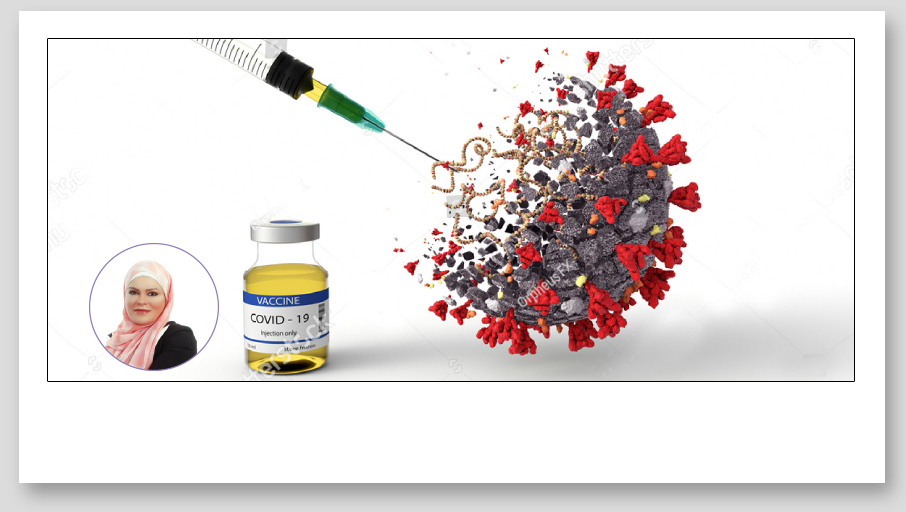COVID-19 Vaccines

This month, we join our medical experts in encouraging the public to get vaccinated. As curfews and lockdowns continue, we are reminded of the severe health risks posed by COVID-19 that often lead to hospitalisation and sometimes death. Our Pharmacist Ruba Al Far answers the most pressing questions she gets asked

To register to receive the COVID-19 vaccine, you can visit the Ministry of Health portal vaccine.jo

 table div table+table+table+table+table+table+table+table+table+table+table+table+table+table+table+table+table+table+table+table+table+table+table+table+table+table+table+table+table+table div table{width:100%;padding:0}table div table+table+table+table+table+table+table+table+table+table+table+table+table+table+table+table+table+table+table+table+table+table+table+table+table+table+table+table+table+table div table img{width:96.23%;padding:0;float:none}table div table+table+table+table+table+table+table+table+table+table+table+table+table+table+table+table+table+table+table+table+table+table+table+table+table+table+table+table+table+table div table td{width:100%;padding:0 1.88% 18px}/* styles */## Our News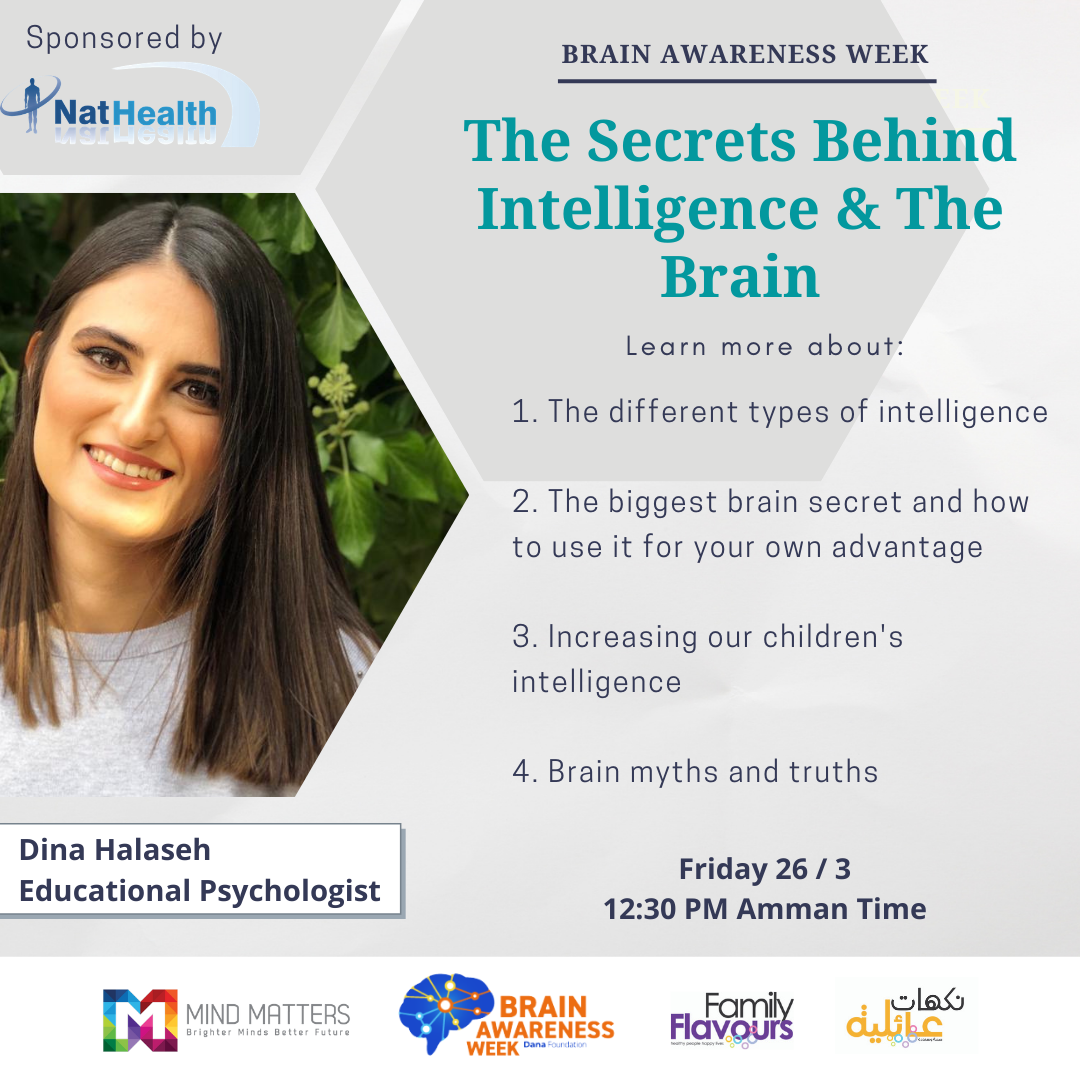Brain Awareness Week
Your brain deserves as much thought and effort to stay healthy as any other part of your life. This is why Al Marji’ Publications partnered with Mind Matters Centre for Brain Awareness Week to share with you the latest research in brain science. The session with our Educational Psychologist Dina Halaseh was sponsored by NatHealth

 table div table+table+table+table+table+table+table+table+table+table+table+table+table+table+table+table+table+table+table+table+table+table+table+table+table+table+table+table+table+table+table+table+table div table{width:100%;padding:0}table div table+table+table+table+table+table+table+table+table+table+table+table+table+table+table+table+table+table+table+table+table+table+table+table+table+table+table+table+table+table+table+table+table div table img{width:96.23%;padding:0;float:none}table div table+table+table+table+table+table+table+table+table+table+table+table+table+table+table+table+table+table+table+table+table+table+table+table+table+table+table+table+table+table+table+table+table div table td{width:100%;padding:0 1.88% 18px}/* styles *//* styles */ Women in Theatre: House of Hope Last March, we featured Seenaryo’s Women’s Theatre Project and the creative work of some 22 Sudanese, Somali, Yemeni and Jordanian women. You can now watch the original theatre piece, House of Hope. The film reflects some of the messages that these women wish to share. Seenaryo is a theatre and play-based learning organisation that works with marginalised and refugee communities in Jordan and Lebanon.
 table div table+table+table+table+table+table+table+table+table+table+table+table+table+table+table+table+table+table+table+table+table+table+table+table+table+table+table+table+table+table+table+table+table+table+table div table{width:100%;padding:0}table div table+table+table+table+table+table+table+table+table+table+table+table+table+table+table+table+table+table+table+table+table+table+table+table+table+table+table+table+table+table+table+table+table+table+table div table img{width:96.23%;padding:0;float:none}table div table+table+table+table+table+table+table+table+table+table+table+table+table+table+table+table+table+table+table+table+table+table+table+table+table+table+table+table+table+table+table+table+table+table+table div table td{width:100%;padding:0 1.88% 18px}/* styles */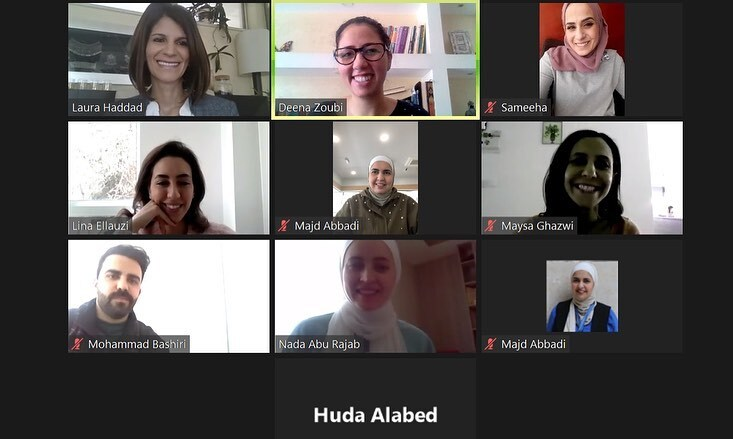Partners in Health & Knowledge
Family Flavours’ Manager-in-Charge Laura Haddad delivered a two-hour writing workshop for the team at the Royal Health Awareness Society (RHAS). The NGO is a longtime health partner, raising awareness through Family Flavours and Nakahat ‘Ailiyeh on health issues that impact families in Jordan

 table div table+table+table+table+table+table+table+table+table+table+table+table+table+table+table+table+table+table+table+table+table+table+table+table+table+table+table+table+table+table+table+table+table+table+table+table+table div table{width:100%;padding:0}table div table+table+table+table+table+table+table+table+table+table+table+table+table+table+table+table+table+table+table+table+table+table+table+table+table+table+table+table+table+table+table+table+table+table+table+table+table div table img{width:96.23%;padding:0;float:none}table div table+table+table+table+table+table+table+table+table+table+table+table+table+table+table+table+table+table+table+table+table+table+table+table+table+table+table+table+table+table+table+table+table+table+table+table+table div table td{width:100%;padding:0 1.88% 18px}/* styles */## This Month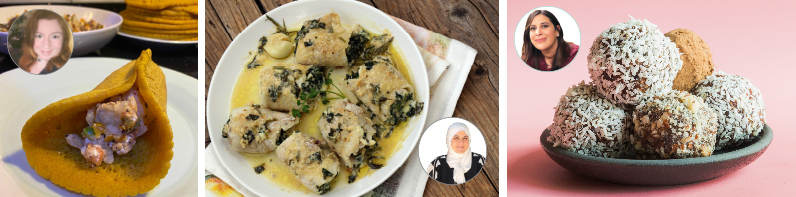/* styles */ Happy Ramadan! If you are celebrating Ramadan, we wish you a healthy and happy Ramadan ahead. Our foodies have been busy preparing an assortment of savoury and sweet dishes for you to give this month a flavourful twist to your regular menu!
 table div table+table+table+table+table+table+table+table+table+table+table+table+table+table+table+table+table+table+table+table+table+table+table+table+table+table+table+table+table+table+table+table+table+table+table+table+table+table+table+table+table div table{width:100%;padding:0}table div table+table+table+table+table+table+table+table+table+table+table+table+table+table+table+table+table+table+table+table+table+table+table+table+table+table+table+table+table+table+table+table+table+table+table+table+table+table+table+table+table div table img{width:96.23%;padding:0;float:none}table div table+table+table+table+table+table+table+table+table+table+table+table+table+table+table+table+table+table+table+table+table+table+table+table+table+table+table+table+table+table+table+table+table+table+table+table+table+table+table+table+table div table td{width:100%;padding:0 1.88% 18px}/* styles */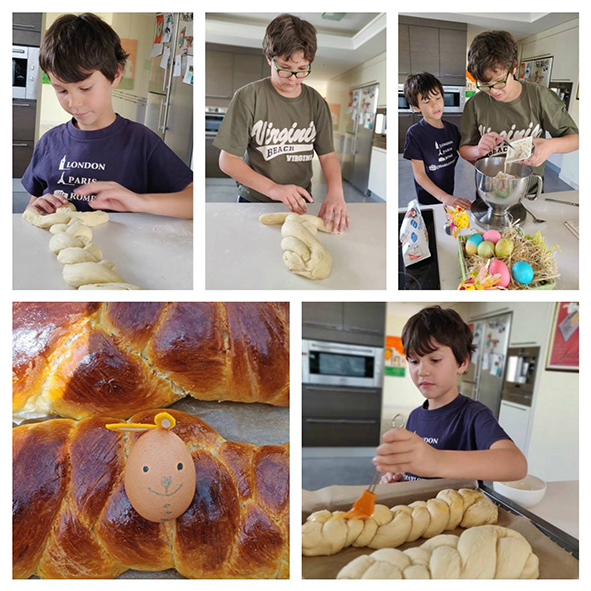Easter Baking
On our pages this month, brothers Omar and Shareef Schwarten share their Teta Virginia’s recipe for semi-sweet braided bread, a family tradition for Easter

 table div table+table+table+table+table+table+table+table+table+table+table+table+table+table+table+table+table+table+table+table+table+table+table+table+table+table+table+table+table+table+table+table+table+table+table+table+table+table+table+table+table+table+table div table{width:100%;padding:0}table div table+table+table+table+table+table+table+table+table+table+table+table+table+table+table+table+table+table+table+table+table+table+table+table+table+table+table+table+table+table+table+table+table+table+table+table+table+table+table+table+table+table+table div table img{width:96.23%;padding:0;float:none}table div table+table+table+table+table+table+table+table+table+table+table+table+table+table+table+table+table+table+table+table+table+table+table+table+table+table+table+table+table+table+table+table+table+table+table+table+table+table+table+table+table+table+table div table td{width:100%;padding:0 1.88% 18px}/* styles */## Here's Your Chance to Win!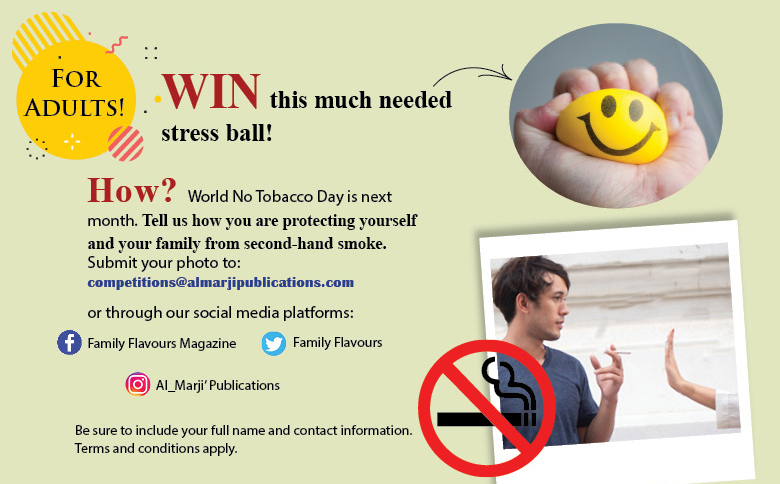table div table+table+table+table+table+table+table+table+table+table+table+table+table+table+table+table+table+table+table+table+table+table+table+table+table+table+table+table+table+table+table+table+table+table+table+table+table+table+table+table+table+table+table+table+table+table div table{width:100%;padding:0}table div table+table+table+table+table+table+table+table+table+table+table+table+table+table+table+table+table+table+table+table+table+table+table+table+table+table+table+table+table+table+table+table+table+table+table+table+table+table+table+table+table+table+table+table+table+table div table img{width:96.23%;padding:0;float:none}table div table+table+table+table+table+table+table+table+table+table+table+table+table+table+table+table+table+table+table+table+table+table+table+table+table+table+table+table+table+table+table+table+table+table+table+table+table+table+table+table+table+table+table+table+table+table div table td{width:100%;padding:0 1.88% 18px}/* styles */table div table+table+table+table+table+table+table+table+table+table+table+table+table+table+table+table+table+table+table+table+table+table+table+table+table+table+table+table+table+table+table+table+table+table+table+table+table+table+table+table+table+table+table+table+table+table+table+table div table{width:100%;padding:0}table div table+table+table+table+table+table+table+table+table+table+table+table+table+table+table+table+table+table+table+table+table+table+table+table+table+table+table+table+table+table+table+table+table+table+table+table+table+table+table+table+table+table+table+table+table+table+table+table div table img{width:96.23%;padding:0;float:none}table div table+table+table+table+table+table+table+table+table+table+table+table+table+table+table+table+table+table+table+table+table+table+table+table+table+table+table+table+table+table+table+table+table+table+table+table+table+table+table+table+table+table+table+table+table+table+table+table div table td{width:100%;padding:0 1.88% 18px}/* styles */## SUBSCRIBE!

 /* styles */ Get Family Flavours delivered directly to your door or inbox! SUBSCRIBE to the online or print versions of the magazine. To ADVERTISE, contact marketing@almarjipublications.com
 table div table+table+table+table+table+table+table+table+table+table+table+table+table+table+table+table+table+table+table+table+table+table+table+table+table+table+table+table+table+table+table+table+table+table+table+table+table+table+table+table+table+table+table+table+table+table+table+table+table+table+table div table{width:100%;padding:0}table div table+table+table+table+table+table+table+table+table+table+table+table+table+table+table+table+table+table+table+table+table+table+table+table+table+table+table+table+table+table+table+table+table+table+table+table+table+table+table+table+table+table+table+table+table+table+table+table+table+table+table div table img{width:96.23%;padding:0;float:none}table div table+table+table+table+table+table+table+table+table+table+table+table+table+table+table+table+table+table+table+table+table+table+table+table+table+table+table+table+table+table+table+table+table+table+table+table+table+table+table+table+table+table+table+table+table+table+table+table+table+table+table div table td{width:100%;padding:0 1.88% 18px}/* styles */Send us an email ourmailbag@almarjipublications.com
Like us on Facebook
Follow us on Twitter
Follow us on Instagram
Follow us on Pinterest
Follow us on Wordpress
Subscribe on YouTube
Add us on Snapchat